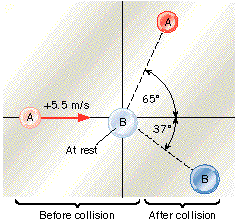# Basic Physics question (2-d momentum)

I can NOT figure this question out, it's the only one I havent gotten, can someone help?

The drawing shows a collision between two pucks on an air-hockey table. Puck A has a mass of 0.020 kg and is moving along the x axis with a velocity of +5.5 m/s. It makes a collision with puck B, which has a mass of 0.040 kg and is initially at rest. The collision is not head-on. After the collision, the two pucks fly apart with the angles shown in the drawing.(a) Find the final speed of puck A.
______ m/s
(b) Find the final speed of puck B.
______ m/s

## Answers and Replies

Hi atlbraves,

usually you must propose a solution or give some hints ........but today I'll make an exception for you:

You must write the the conservation law for angular momentum:

$$\vec{p_{A0}}=\vec{p_A}+\vec{p_B}$$

Then you have to write the above vectorial equation on Ox (the direction of the initial velocity of A) and Oy (perpendicular on it):

Ox: $$p_{A0}=p_A \cdot cos(\alpha_A)+p_B \cdot cos(\alpha_B)$$
Oy: $$p_A \cdot sin(\alpha_A)-p_B \cdot sin(\alpha_B)=0$$

clive said:
Hi atlbraves,

usually you must propose a solution or give some hints ........but today I'll make an exception for you:

You must write the the conservation law for angular momentum:

$$\vec{p_{A0}}=\vec{p_A}+\vec{p_B}$$

Then you have to write the above vectorial equation on Ox (the direction of the initial velocity of A) and Oy (perpendicular on it):

Ox: $$p_{A0}=p_A \cdot cos(\alpha_A)+p_B \cdot cos(\alpha_B)$$
Oy: $$p_A \cdot sin(\alpha_A)-p_B \cdot sin(\alpha_B)=0$$

thanks, i figured it out, i actually ended up knowing how to do it, the reason i wasnt getting the answer correct is i messed up a calculation, thanks for the help anyways